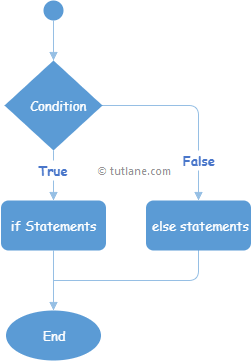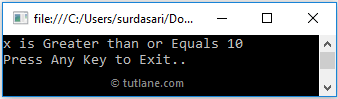# Visual Basic If Else Statement

In Visual Basic, If Else statement or condition has an optional Else statements, the Else statements will be executed whenever the If condition fails to execute.

Generally in Visual Basic, If Else statement, whenever the boolean expression returns true, the If statements will be executed; otherwise, the Else block of statements will be executed.

## Syntax of Visual Basic If Else Statement

Following is the syntax of defining the If Else statement in Visual Basic programming language.

If boolean_expression Then
// Statements to Execute if boolean expression is True
Else
// Statements to Execute if boolean expression is False
End If

If you observe the above If Else statement syntax, the statements inside the If condition will be executed only when the “bool_expression” returns true otherwise, the statements inside of Else condition will be executed.

Following is the sample example of using the If Else statement in a visual basic programming language.

Dim x As Integer = 20
If x >= 10 Then
Console.WriteLine("x is Greater than or equals 10")
Else
Console.WriteLine("x is less than or equals to 10")
End If

If you observe the above example, whenever the defined condition (x >= 10) returns true, the If condition will be executed; otherwise, the Else block of code will be executed.

## Visual Basic If Else Statement Flow Chart

Following is the flow chart diagram, representing the process flow of the If Else statement in Visual Basic programming language.If you observe the above Visual Basic If Else statement flow chart diagram when the defined condition is true, the statements within the If condition will be executed; otherwise, the statements within the Else block will be executed.

## Visual Basic If Else Statement Example

Following is the example of defining the If Else statement in Visual Basic programming language to execute the block of code or statements based on a Boolean expression.

Module Module1
Sub Main()
Dim x As Integer = 20
If x >= 10 Then
Console.WriteLine("x is Greater than or Equals to 10")
Else
Console.WriteLine("x is Less than 10")
End If
Console.WriteLine("Press Enter Key to Exit..")
End Sub
End Module

If you observe the above example, we defined the If-Else condition to execute the statements based on the defined condition status i.e. either true or false.

When we execute the above Visual Basic program, we will get the result as shown below.If you observe the above result, the Boolean expression returns true that’s the reason only If block of statements has been executed and printed the statements in the console window.

This is how we can use the If Else statement in Visual Basic programming language to execute the block of code or statements based on our requirements.